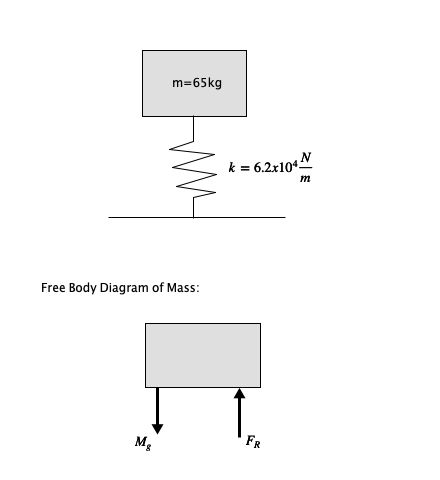# If a 65\ \mathrm{kg} mass drops vertically onto a spring with stiffness coefficient of 6.2\times...

## Question:

If a {eq}65\ \mathrm{kg} {/eq} mass drops vertically onto a spring with stiffness coefficient of {eq}6.2\times 10^4\ \mathrm{N/m} {/eq}, how far does it depress the spring?

## Stiffness Coefficient:

A spring's stiffness coefficient, also known as the spring constant is the amount of force (in Newtons) required to depress or stretch a spring one meter from its equilibrium position. Another way to look at the stiffness coefficient is to look at Hooke's law. Hooke's law defines the force a spring exerts on an object as:

{eq}F=-kx {/eq}

Where k is the stiffness coefficient and x is the distance that the spring is being displaced. The minus sign shows that the force the spring exerts (F) is in the opposite direction that the spring is being displaced from its equilibrium position (x).

Another concept that will be used in the answering of this question is Newton's second law, which states that force is equal to mass times acceleration. For a falling object, the force of the object due to gravity ({eq}M_g {/eq}) is equal to the mass of the object (m) times the acceleration due to gravity {eq}g=-9.81\frac{m}{s^2} {/eq}:

{eq}M_g = mg {/eq}

Become a Study.com member to unlock this answer! Create your account

First, let us draw what is occurring in this problem, including a free body diagram of the dropped mass:We can calculate the force on the mass...Hooke's Law & the Spring Constant: Definition & Equation

from

Chapter 4 / Lesson 19
201K

After watching this video, you will be able to explain what Hooke's Law is and use the equation for Hooke's Law to solve problems. A short quiz will follow.